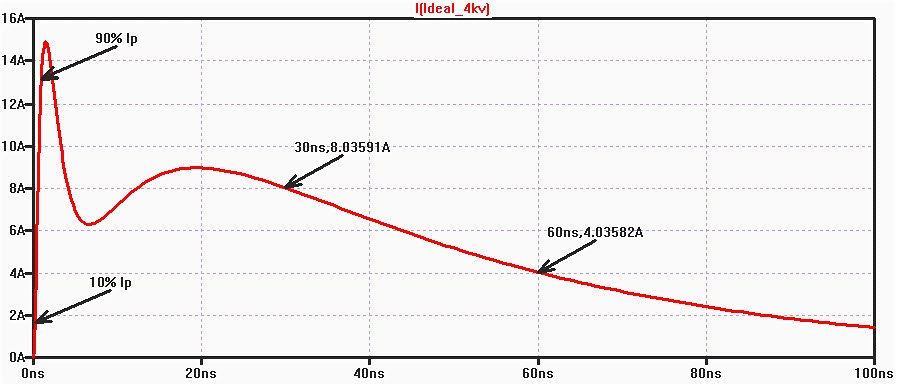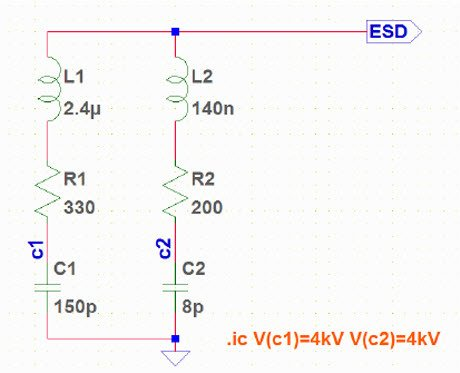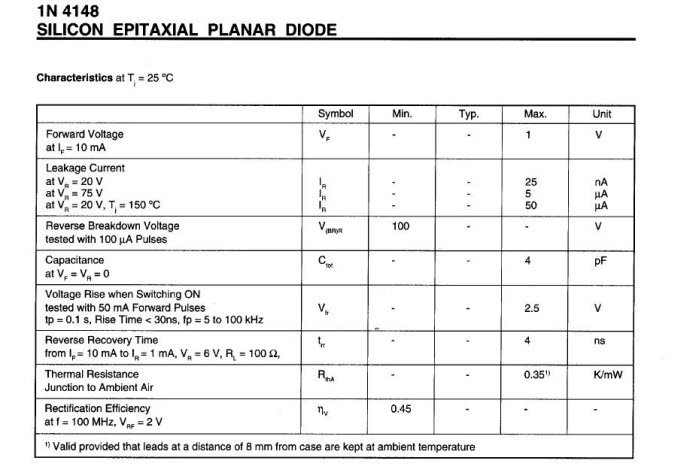# TINA Design Suite Video tutorials

TopicVideo
Introductio to TINA Design Suite
Comparison of simulation and real time measurements
Real time transient recording and comparison with simulation
RC transient and AC analysys
Fourier analysis
Active band pass filter
How to make subcircuits from schematics
Test a logic gate in interactive mode
Bus example
Pic flasher circuit
VHDL circuits
Create a simple PCB
Importing PSpice Netlists into TINA

# Simple SPICE ESD Generator Circuit based on IEC61000-4-2 Standard

Università Politecnica delle Marche, Ancona, Italy.

by

Ing. Luca Buccolini

#### WHAT IS ESD?

Static charge is an unbalanced electrical charge at rest. Typically, it is created by insulator surfaces rubbing together or pulling apart. One surface gains electrons, while the other surface loses electrons. This results in an unbalanced electrical condition known as static charge.

When a static charge moves from one surface to another, it becomes ESD. ESD is a miniature lightning bolt of charge that moves between two surfaces that have different potentials. It can occur only when the voltage differential between the two surfaces is sufficiently high to break down the dielectric strength of the medium separating the two surfaces.

When a static charge moves, it becomes a current that damages or destroys gate oxide, metallization, and junctions. ESD can occur in any one of four different ways: a charged body can touch an IC, a charged IC can touch a grounded surface, a charged machine can touch an IC, or an electrostatic field can induce a voltage across a dielectric sufficient to break it down

#### ESD STRESS MODELS

ESD can have serious detrimental effects on all semiconductor ICs and the system that contains them. Standards are developed to enhance the quality and reliability of ICs by ensuring all devices employed have undergone proper ESD design and testing, thereby, minimizing the detrimental effects of ESD. Three major stress methods are widely used in the industry today to describe uniform methods for establishing ESD withstand thresholds (highest passing level).

#### HUMAN BODY MODEL (HBM)

The HBM is a component level stress developed to simulate the action of a human body discharging accumulated static charge through a device to ground, and employs a series RC network consisting of a 100 pF capacitor and a 1500 Ohm resistor.

#### CHARGED DEVICE MODEL (CDM)

The CDM is a component level stress that simulates charging and discharging events that occur in production equipment and processes. Potential for CDM ESD events occur when there is metal-to-metal contact in manufacturing.

#### SYSTEM LEVEL ESD (IEC 61000-4-2)

The IEC system level ESD is a widely accepted European standard that defines an ESD event that is meant to be tested on actual end equipment to simulate a charged person or object discharging into electronic systems. The IEC standard defines an ESD stress that is much stronger than the component level ESD stresses defined by HBM and CDM.

The engineer must design following IEC 61000-4-2 standard to be able to declare the conformity CE (“Conformité Européenne”).

The ESD generator circuit realized in this work is compliant with ESD generator used in EMC laboratories to perform CE-conformity tests, thus a SPICE simulation can be used to test ESD-immunity solutions before circuit production.

#### IEC 61000-4-2 WAVEFORM CHARACTERISTICS

The standard accurately describe the characteristics and performances of the ESD generator as well as the current waveform parameters.

The ESD phenomenon is a very short but very strong current transient and is represented in Figure 1.Figure 1. 61000-4-2 ideal contact ESD waveform at 4kV

This pulse is divided into two parts: The first peak, known as the “Initial Peak”, is caused by the discharge of the arm, and generates the maximum current. The second peak is caused by the discharge of the body. The rise time of the initial peak is between 0.6 ns and 1 ns, and its amplitude depends on the charging voltage of the ESD simulator.

The standard describe a formula and the mains current level of the waveform for different test level.Table 1 contact discharge current waveform parameters

Even though the IEC 61000-4-2  include a simplified circuit of ESD generator, it is incompatible with the discharge current equation descripted in the standard and the waveform shown in Figure 1. A PSpice software simulation can prove this.

#### THE BASIC ESD GENERATOR MODEL

In order to run ESD stress simulation, an ESD-generator model was built. This can help engineers to test different solutions in a SPICE simulator to overcome strength over-voltages before realizing PCB circuit and test it against ESD.

The objective is to generate an ESD pulse that accurately corresponds to the current stress waveforms at various stress levels in accordance with the IEC 61000-4-2 specification.

The ESD can be simulated by two parallel R, L, C circuits with charged capacitors. The generator equivalent circuit is shown in Figure 2.

Here the standardized network elements of the ESD-generator are represented by R1 (330ohm) and C1 (150pF). The inductor L1 is considered to be the obligatory ground strap with the length of about 2 m. Physically the first peak of the pulse is shaped by additional lumped and parasitic elements around and in the tip of the ESD-generator .

Note that the values of R, L, and C for both branches are tweaked to correctly represent standard IEC stress waveform; “.ic V(c1)=4kV V(c2)=4kV” refers to the initial condition of the voltage on the capacitors for a 4kV zap.Figure 2  The general equivalent circuit of basic IEC61000-4-2 generator model

# SPICE modeling of a Diode from Datasheet

Modeling in SPICE& a diode is not a trivial work. Although the operation of the diode is quite simple, extract a model from datasheet takes some time.Every component has its own syntax defined in SPICE , in the case of the diode:

.model ModelName D (par1=a par2=b………parn=x)

where par1 par2 …. parn are characteristic parameters of diode.

we can sum up the set of main parameters in the following table:

ParameterDescriptionUnitDefault value
BVReverse breakdown knee voltageVInfinite
CJOZero-bias p-n capacitanceF0
EGBandgap voltageeV1.11
FCForward-bias depletion capacitance coefficientno unit dimension0.5
IBLVLow-level reverse breakdown knee correntA0
IBVReverse breakdown knee correntA1e-10
IKFHigh-injection knee currentAInfinite
ISSaturation correntA1e-14
ISRRecombination current parameterA0
NEmission coefficientno unit dimension1.0
NREmission coefficient for ISRno unit dimension2.0
RSParasitic resistanceOhm0
TTTransit timesec0
VJp-n potentialV1.0
XTIIS temperature exponentno unit dimension3.0

All these parameters are used by SPICE to describe the behavior of the diode in the different situations of signal, for example in direct polarization in DC that, forward current will be:

ID = IS*(e^(VD/(N*Vt))-1)

where VD is the forward voltage, Vt = k * T / q is the thermal voltage equal to 0.026 V at 27 degrees Celsius.

The so-called recombination current is instead calculated as

Irec = ISR*(e^(VD/(N*Vt))-1).

Other equations from the given parameters describing the capacitance of the junction, its evolution with temperature and more.

At this point we have to derive the various parameters from the datasheet of the component. Assume we want to model a silicon diode 1N4148. The extraction of the parameters of the table from the values reported in the datasheet, is not immediate for almost none of the parameters.look at the values of our interest in datasheet:From the table we can get BV which is equal to VRM, in other cases reported as Vbr, or in the case of Zener diode Vz.From this second table we see that the maximum leakage current at 25 degrees is Ir = 5 uA.We can take IBV as equal to 10 times Ir. Usually for this type of diodes the value of IBV is around 100uA. For Zener diodes Ir can be called Izk, or in other cases as Ibr.

CJO can be directly equal to the value specified in the datasheet as Cj or Ctot, in this case is 4pF.